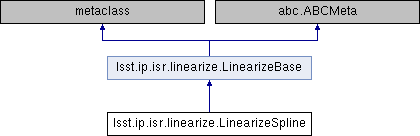lsst.ip.isr.linearize.LinearizeSpline Class Reference
Inheritance diagram for lsst.ip.isr.linearize.LinearizeSpline:## Public Member Functions

def __call__ (self, image, **kwargs)

## Static Public Attributes

string LinearityType = "Spline"

## Detailed Description

Correct non-linearity with a spline model.

corrImage = uncorrImage - Spline(coeffs, uncorrImage)

Notes
-----

The spline fit calculates a correction as a function of the
expected linear flux term.  Because of this, the correction needs
to be subtracted from the observed flux.

Definition at line 690 of file linearize.py.

## ◆ __call__()

 def lsst.ip.isr.linearize.LinearizeSpline.__call__ ( self, image, ** kwargs )
Correct for non-linearity.

Parameters
----------
image : lsst.afw.image.Image
Image to be corrected
kwargs : dict
Dictionary of parameter keywords:
"coeffs"
Coefficient vector (list or numpy.array).
"log"
Logger to handle messages (logging.Logger).

Returns
-------
output : tuple [bool, int]
If true, a correction was applied successfully.  The
integer indicates the number of pixels that were
uncorrectable by being out of range.


Reimplemented from lsst.ip.isr.linearize.LinearizeBase.

Definition at line 705 of file linearize.py.

705  def __call__(self, image, **kwargs):
706  """Correct for non-linearity.
707
708  Parameters
709  ----------
710  image : lsst.afw.image.Image
711  Image to be corrected
712  kwargs : dict
713  Dictionary of parameter keywords:
714  "coeffs"
715  Coefficient vector (list or numpy.array).
716  "log"
717  Logger to handle messages (logging.Logger).
718
719  Returns
720  -------
721  output : tuple [bool, int]
722  If true, a correction was applied successfully. The
723  integer indicates the number of pixels that were
724  uncorrectable by being out of range.
725  """
726  splineCoeff = kwargs['coeffs']
727  centers, values = np.split(splineCoeff, 2)
728  interp = afwMath.makeInterpolate(centers.tolist(), values.tolist(),
729  afwMath.stringToInterpStyle("AKIMA_SPLINE"))
730
731  ampArr = image.getArray()
732  delta = interp.interpolate(ampArr.flatten())
733  ampArr -= np.array(delta).reshape(ampArr.shape)
734
735  return True, 0
736
737
Interpolate::Style stringToInterpStyle(std::string const &style)
Conversion function to switch a string to an Interpolate::Style.
Definition: Interpolate.cc:256
std::shared_ptr< Interpolate > makeInterpolate(std::vector< double > const &x, std::vector< double > const &y, Interpolate::Style const style=Interpolate::AKIMA_SPLINE)
A factory function to make Interpolate objects.
Definition: Interpolate.cc:342

## ◆ LinearityType

 string lsst.ip.isr.linearize.LinearizeSpline.LinearityType = "Spline"
static

Definition at line 703 of file linearize.py.

The documentation for this class was generated from the following file:
• /j/snowflake/release/lsstsw/stack/lsst-scipipe-0.7.0/Linux64/ip_isr/22.0.1-27-g97737f7+2a20fdde0d/python/lsst/ip/isr/linearize.py